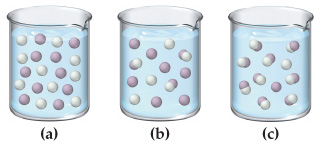⚠️Our tutors found the solution shown to be helpful for the problem you're searching for. We don't have the exact solution yet.

# Solution: The following three diagrams represent three different solutions of the binary acid HA. Water molecules have been omitted for clarity and hydronium ions (H3O+) are represented by hydrogen ions (H+). Rank the acids in order of decreasing acid strength.

###### Problem

The following three diagrams represent three different solutions of the binary acid HA. Water molecules have been omitted for clarity and hydronium ions (H3O+) are represented by hydrogen ions (H+). Rank the acids in order of decreasing acid strength.